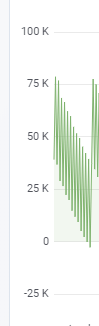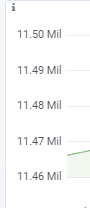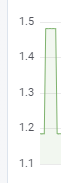# Why auto decimals?

decimals指的是传统意义上的保留小数位，如1.23对应的就是2。

scaledDecimals指的则是单位转换过后的保留小数位，比如kbn转换时间时会默认将超过1000的时间进位，1234ms -> 1.23s，这里1.23就是scaledDecimals = -1时的结果（单位转换时会增加decimals，这里从ms -> s就增加了3位decimals）。

decimals和scaledDecimals的使用规则是，如果没有scaledDecimals统一使用decimals，有的话在发生单位转换时使用scaledDecimals，其他情况使用decimals。

# grafana的auto decimals算法export function getFlotTickDecimals(datamin, datamax, axis, height) {
const { min, max } = getFlotRange(axis.min, axis.max, datamin, datamax);
const noTicks = 0.3 * Math.sqrt(height);
const delta = (max - min) / noTicks;
const dec = -Math.floor(Math.log(delta) / Math.LN10);

const magn = Math.pow(10, -dec);
// norm is between 1.0 and 10.0
const norm = delta / magn;
let size;

if (norm < 1.5) {
size = 1;
} else if (norm < 3) {
size = 2;
// special case for 2.5, requires an extra decimal
if (norm > 2.25) {
size = 2.5;
}
} else if (norm < 7.5) {
size = 5;
} else {
size = 10;
}
size *= magn;

const tickDecimals = Math.max(0, -Math.floor(Math.log(delta) / Math.LN10) + 1);
const scaledDecimals = tickDecimals - Math.floor(Math.log(size) / Math.LN10);
return { tickDecimals, scaledDecimals };
}